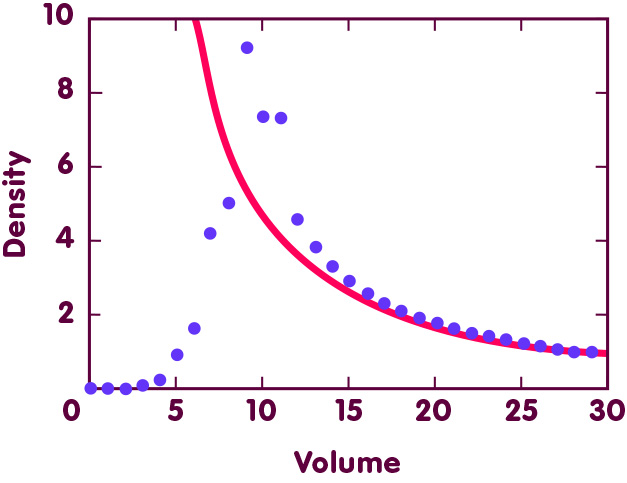# Taming infinity with loops

### How loop quantum gravity could replace the absurd state of infinite density, the big bang with which, according to Einstein’s relativity, the universe began

#### An article by Martin Bojowald

In the conventional big bang models based on general relativity, our universe began in a patently absurd physical state: With the big bang singularity, at a time when all the space and matter we see around us today was compressed to a single point of infinitely high density. Such infinities are a sure sign for “pathological” physics; a sure sign that Einstein’s equations which, in the big bang models, govern the evolution of the cosmos, lose their meaning directly at the big bang.

For decades, there has been hope that a theory unifying general relativity with the concepts of quantum theory (in short, a theory of quantum gravity) would solve the problem of these infinities. These hopes have recently taken on a more concrete shape, in the framework of so-called loop quantum gravity and its applications to cosmology.

## Quanta of space and time

In this theory, there is a kind of quantization of the structure of space and time. In Einstein’s theory, space is a continuum: every region of space can be subdivided further. In loop quantum gravity, matters are different: there, every length is a multiple of an elementary length called the Planck length. Two points in space can have a distance of one, two, three or more Planck length, but they cannot be one and a half, or two-thirds of a Planck length apart. Length is not arbitrarily divisible – there is an ultimate subdivision, that into the minimal units of length.

The Planck length is extremely small – around 10-35 metres, and hence far beyond the microscopic distances that can be explored with our most powerful “microscopes”: the world’s largest particle accelerators. That’s why the subdivision of length into fixed minimal building blocks – its “quantization” – is irrelevant for everyday life, and the same goes for most of physics. However, according to the big bang models, roughly 14 billion years ago, the whole of the observable universe was confined to a space a few Planck lengths in diameter – and at that point in time, close to the big bang, the quantum structure of space and time becomes important indeed.

## Avoiding infinite density

As it turns out, the quantization of space as predicted by loop quantum gravity promises to fulfill physicists’ hopes of a cosmology that is free of infinities. In ordinary physics, these infinities are inescapable: there, the average density in a region of space is equal to the mass contained in that region, divided by the region’s volume. If that region contracts to zero volume, the density becomes infinite. In loop quantum gravity, the relation between density and volume is more complicated, as far as very small volumes are concerned. The following image shows the density of a fixed amount of energy, confined to smaller and smaller volumes, as predicted by loop quantum gravity:Volume is plotted on the horizontal axis, density on the vertical axis. The red, solid line shows the evolution of density in classical physics, for instance in general relativity. The voilet dots show the density values predicted by loop quantum gravity for matter that is, to the far right, confined to 30 times the elementary volume, then to a mere 29, and so on, until, on the left, space has the volume of a single elementary cube – Planck length cubed – and, finally, space contracts to volume zero. The crucial feature: as space shrinks, density can take on large values, but it does not become infinite, even when space shrinks to a point.

In this way, the ugly big bang singularity with its infinite density can be eliminated. Instead, in cosmological models based on loop quantum gravity, there is history before the big bang. The evolution of the universe is governed by its energy density. The non-classical dependence of such densities on volume corresponds to a repulsive force at very small scales. This effect clearly shows the difference between quantum gravity and ordinary general relativity – in relativity, gravity is always attractive. Thanks to this repulsive force, the quantized Einstein equations can describe physics at the big bang, and beyond – a clear advantage over general relativity. (More informations about this modified evolution can be found in the spotlight topic Avoiding the big bang.)

## Further Information

###### Colophon
Martin Bojowald

is a physics professor at Pennsylvania State University with a research focus on cosmological applications of loop quantum gravity.